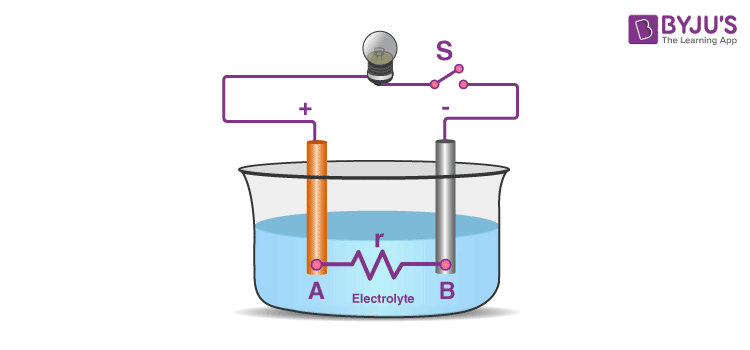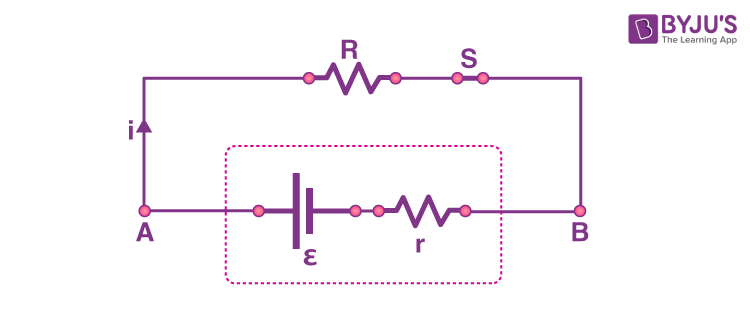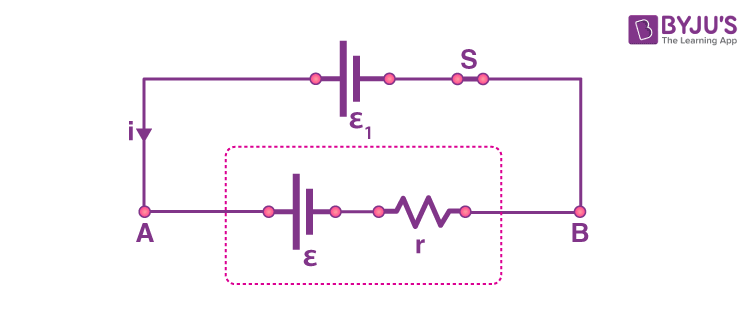# Electric Cell

The simple device to maintain an electric current in a circuit is called the electric cell. Cells provide electric energy through chemical reactions. Basically, the cell has two electrodes, called the positive and negative. These electrodes are immersed in the electrolytic solution. When we look at the cell we would notice that the positive terminal of the cell has a metal cap and the negative terminal has a flat metal disc. When the positive and the negative terminals are connected to the electrical device, the current starts flowing. Internal reactions happen in the chemicals present inside the cell to produce electricity. The chemicals get used up over time and the cell cannot produce electric energy anymore. A group of different cells is called a battery.

## Electric Cell – Electromotive Force (EMF)The electric cell can be defined as an external source that creates a potential difference between two points A and B in the circuit. The cell will create a potential difference between the terminals A and B that is equal to the EMF (Electromotive force) of the cell when it is not connected to the external circuit or we can say when the circuit is an open circuit without any flow of current. The work done in driving a unit charge across the terminals is called electromotive force. EMF is not a force, it is the potential difference. The unit of EMF is volt.

The electromotive force is denoted as ε.

ε = W/q = VAB

here,

W is the work done

q is the unit charge

VAB is the potential difference across terminals A and B

## Internal Resistance and EMF

### Case 1: When the cell acts as a sourceDischarging

There will be resistance to the flow of current due to the material of the cell. This is called internal resistance. It is measured in ohms.

Let us consider that the cell acting as the source of current is connected to an external circuit (closed circuit). Due to the internal resistance when the cell gives out current (discharging) the measured potential difference across terminals A and B will be less than the actual EMF of the cell

VAB = ε – ir

Here

VAB is called the terminal voltage or potential difference across terminals A and B

ε is the EMF of the cell

r is the internal resistance of the cell

i is the current through the circuit

ir is the voltage drop due to the internal resistance

The negative sign in the expression is because the current flows from the positive terminal A to the negative terminal B. From the above expression, we understand that the terminal voltage is less than the EMF of the cell when the current flows out of the positive terminal of the cell.

### Case 2: When the cell acts as the loadCharging

When the cell is charging it acts as a load. In this case, the current enters the positive terminal of the cell. Therefore, the terminal voltage will be

VAB = ε + ir

Here

VAB is called the terminal voltage

ε is the EMF of the cell

r is the internal resistance of the cell

i is the current through the circuit

The terminal voltage is greater than the EMF of the cell when the current enters the positive terminal of the cell.

### Solved Examples

1. In an open circuit condition, the terminal voltage will be

a) equal to the EMF of the cell

b) more than the EMF of the cell

c) less than the EMF of the cell

d) Changes with internal resistance

Answer: a) equal to the EMF of the cell

Solution:

The terminal voltage and the EMF of the cell will be equal since there will not be any effect due to the internal resistance in an open circuit.

2. The unit of EMF is

a) Volt

b) Joule

c) Ampere

d) Dyne

Solution:

The unit of EMF is the same as that of voltage. Therefore, it is volt.

3. The potential difference across the terminals of the cell is equal to 3 volts when there is no current flow through the circuit. The potential difference falls to 2.8 volts when a cell is connected to the external circuit. Find the internal resistance of the cell.

Solution:

V = ε – ir

⇒ r = (ε – V)/r

= (3 – 2.8)/0.37

= 0.54 Ω

Related Video